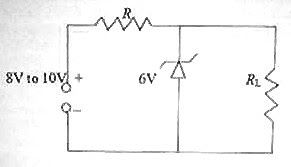## Sunday, June 22, 2008

### Electronics: Multiple Choice Questions on Zener Diodes

In electronics, occasionally you will get questions meant for high lighting the principle of operation and use of Zener diodes. Questions in this section are usually simple. Consider the following question which appeared in KEAM (Medical) 2008 question paper:

In the given circuit, the current through the resistor 2 kΩ is(a) 2 mA

(b) 4 mA

(c) 6 mA

(d) 1 mA

(e) 10 mA

This is a very simple question. The voltage across the reverse biased Zener diode is its breakdown voltage, which is indicated in the figure as 12 V. The load resistor 2 kΩ is connected across the Zener diode and hence the current through it is 12 V/2 kΩ = 6 mA.

Now consider the following question pertaining to the points you have to remember in using a Zener diode as a simple shunt voltage regulator (of the type in the above question):

In the simple shunt voltage regulator circuit shown in the adjoining figure, the unregulated supply voltage varies between 8 V and 10 V. If the maximum reverse current that the Zener diode can safely handle is 100 mA and the Zener diode should draw a minimum current of 5mA (for reliable operation of the voltage regulator), what is the expected maximum current drawn by the load resistor RL?

(a) 95 mA

(b) 105 mA

(c) 200 mA

(d) 10 mA

(e) 110 mA

Since the maximum current allowed through the Zener diode is 100 mA, the maximum current drawn by the Zener diode and the load resistor together is 100 mA itself. If the load is disconnected, the entire current will flow through the Zener diode and this is to be limited to 100 mA.

As the Zener diode is expected to draw at least 5 mA (which happens when the load draws the maximum current), the maximum expected load current is (100 mA – 5 mA) = 95 mA.

[Note that in the shunt voltage regulator, the Zener diode and the load together draws the same current irrespective of the input voltage variation and the load current variation (within the design limits)].

In the above question, what is the value of the series resistance R?

(a) 10 Ω

(b) 20 Ω

(c) 40 Ω

(d) 60 Ω

(e) 95 Ω

You have to consider the maximum unregulated input voltage to determine the value of R. Here it is 10 V. Since the regulated voltage (break down voltage of the Zener diode) is 6 V, the voltage drop across R must be 10 V– 6 V = 4 V.

Since the current through R in all situations (within design limits) is 100 mA, the value of R is given by

R = 4 V/ 100 mA = 4 V/ 0.1 A = 40 Ω.

1.2.